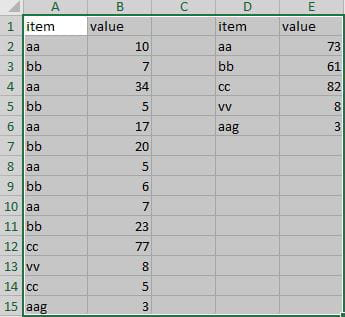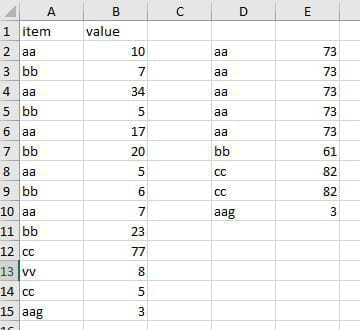# Modified code sum repeat data

Solved/Closed
abdelfatah_0230 Posts 73 Registration date Thursday July 18, 2019 Status Member Last seen July 23, 2022 - Updated on Oct 8, 2020 at 11:42 AM
abdelfatah_0230 Posts 73 Registration date Thursday July 18, 2019 Status Member Last seen July 23, 2022 - Oct 27, 2020 at 06:11 PM
hello
actually i search code to sum repeat values and i have about 2000 rows so that's why i do that by code instead of formula the result not right
the image 1 is the right result in col d,e
the image 2 is wrong what i got after run codeand this my code
`Option ExplicitSub Main()    CollectArray "A", "D"    DoSum "D", "E", "A", "B"End Sub' collect array from a specific column and print it to a new one without duplicates' params:'           fromColumn - this is the column you need to remove duplicates from'           toColumn - this will reprint the array without the duplicatesSub CollectArray(fromColumn As String, toColumn As String)    ReDim arr(1) As String    Dim i As Long    For i = 2 To Range(fromColumn & Rows.Count).End(xlUp).Row        arr(UBound(arr)) = Range(fromColumn & i)        ReDim Preserve arr(UBound(arr) + 1)    Next i    ReDim Preserve arr(UBound(arr) - 1)    RemoveDuplicate arr    Range(toColumn & "1:" & toColumn & Range(toColumn & Rows.Count).End(xlUp).Row).ClearContents    For i = LBound(arr) To UBound(arr)        Range(toColumn & i + 1) = arr(i)    Next iEnd Sub'' params:'           fromColumn - this is the column with string to match against'           toColumn - this is where the SUM will be printed to'           originalColumn - this is the original column including duplicate'           valueColumn - this is the column with the values to sumPrivate Sub DoSum(fromColumn As String, toColumn As String, originalColumn As String, valueColumn As String)    Range(toColumn & "1:" & toColumn & Range(toColumn & Rows.Count).End(xlUp).Row).ClearContents    Dim i As Long    For i = 2 To Range(fromColumn & Rows.Count).End(xlUp).Row        Range(toColumn & i) = WorksheetFunction.SumIf(Range(originalColumn & ":" & originalColumn), Range(fromColumn & i), Range(valueColumn & ":" & valueColumn))    Next iEnd SubPrivate Sub RemoveDuplicate(ByRef StringArray() As String)    Dim lowBound\$, UpBound&, A&, B&, cur&, tempArray() As String    If (Not StringArray) = True Then Exit Sub    lowBound = LBound(StringArray): UpBound = UBound(StringArray)    ReDim tempArray(lowBound To UpBound)    cur = lowBound: tempArray(cur) = StringArray(lowBound)    For A = lowBound + 1 To UpBound        For B = lowBound To cur            If LenB(tempArray(B)) = LenB(StringArray(A)) Then                If InStrB(1, StringArray(A), tempArray(B), vbBinaryCompare) = 1 Then Exit For            End If        Next B        If B > cur Then cur = B        tempArray(cur) = StringArray(A)    Next A    ReDim Preserve tempArray(lowBound To cur): StringArray = tempArrayEnd Sub`

Related:

## 3 responses

TrowaD Posts 2921 Registration date Sunday September 12, 2010 Status Moderator Last seen January 16, 2023 549
Oct 12, 2020 at 12:01 PM
Hi Abdel,

Why would a formula produce an incorrect result? Have you tried this one:
=SUMIF(A:A,D2,B:B)

Best regards,
Trowa
abdelfatah_0230 Posts 73 Registration date Thursday July 18, 2019 Status Member Last seen July 23, 2022
Oct 17, 2020 at 06:21 AM
hi, Trowa sorry about delaying my answer about the formula i know it but i have ever told you contain a huge data that's why i would do that by code it takes more time to pull the formula down about 2000 rows if can you fix my code or alternative to do that i truly appreciate that
thanks again
TrowaD Posts 2921 Registration date Sunday September 12, 2010 Status Moderator Last seen January 16, 2023 549
Oct 26, 2020 at 12:37 PM
Hi Abdel,

Your code looks overly complicated, so I decided to write my own. Here is my take on getting the requested task done:
```Sub RunMe()
Dim mFind As Range

Columns("A:A").Copy Columns("D:D")

For Each cell In Range("D2:D" & Range("D1").End(xlDown).Row)
Set mFind = Columns("A:A").Find(what:=cell.Value, lookat:=xlWhole)
Do
cell.Offset(0, 1).Value = cell.Offset(0, 1).Value + mFind.Offset(0, 1).Value
Set mFind = Columns("A:A").FindNext(mFind)
Next cell
End Sub```

Best regards,
Trowa
abdelfatah_0230 Posts 73 Registration date Thursday July 18, 2019 Status Member Last seen July 23, 2022
Oct 26, 2020 at 04:22 PM
thanks Trowa but your code doesn't work well it sums values repeatedly each item it continues summing every time run the macro it supposing summing one if i time even run macro repeatedly
TrowaD Posts 2921 Registration date Sunday September 12, 2010 Status Moderator Last seen January 16, 2023 549
Oct 27, 2020 at 12:33 PM
Hi Abdel,

When you want to run the code multiple times, then you can clear the results first:
`Range("D2:E" & Range("E1").End(xlDown).Row).ClearContents`

Or use the slightly adjusted code below:
```Sub RunMe()
Dim mCount As Long
Dim mFind As Range

Columns("A:A").Copy Columns("D:D")

For Each cell In Range("D2:D" & Range("D1").End(xlDown).Row)
Set mFind = Columns("A:A").Find(what:=cell.Value, lookat:=xlWhole)
Do
mCount = mCount + mFind.Offset(0, 1).Value
Set mFind = Columns("A:A").FindNext(mFind)